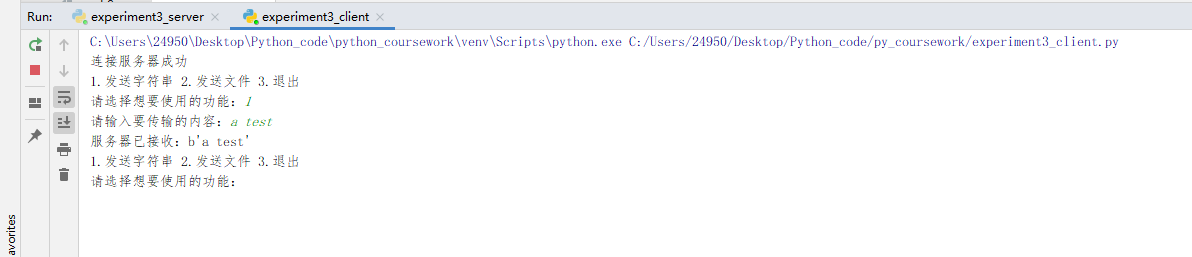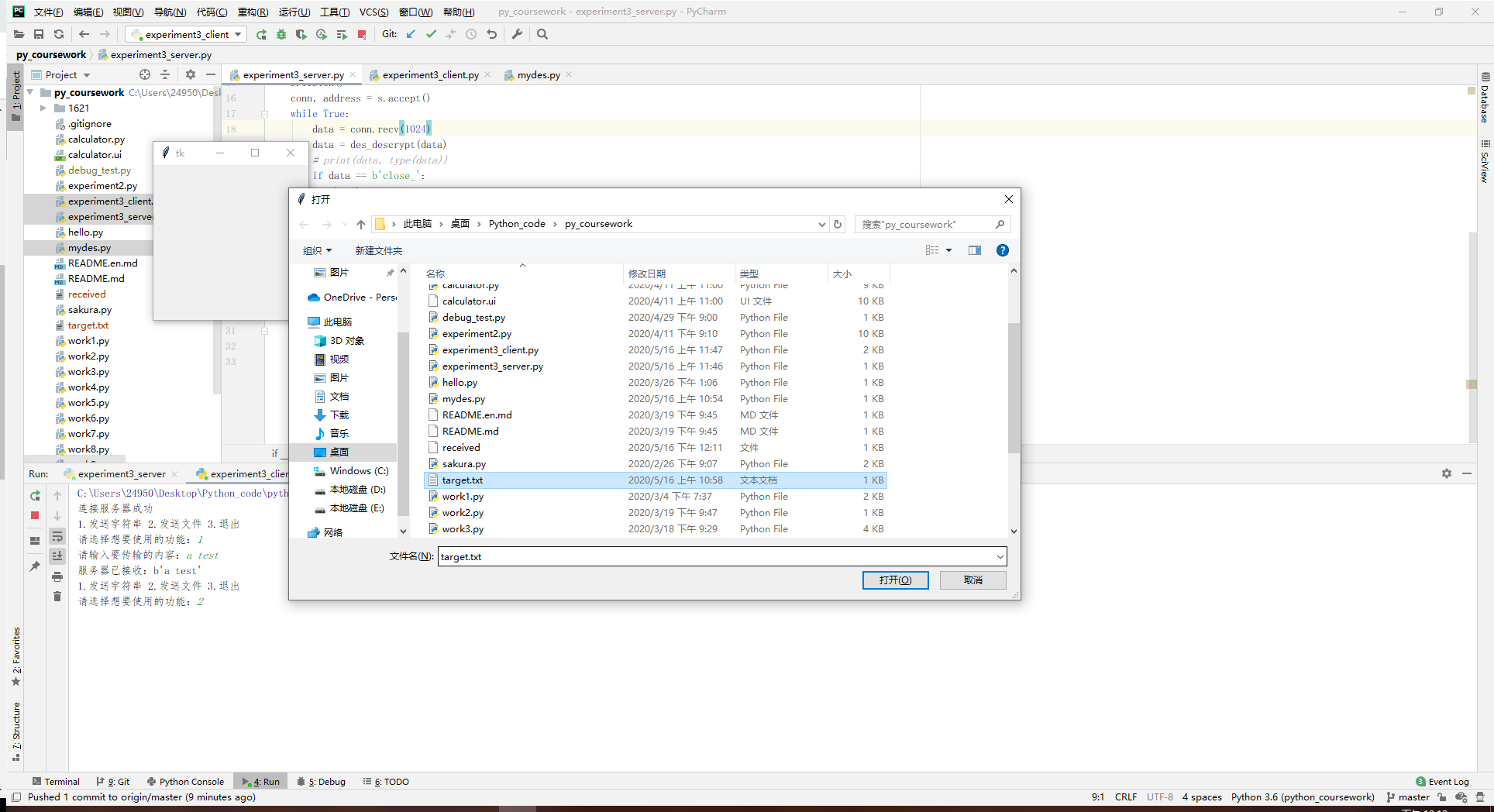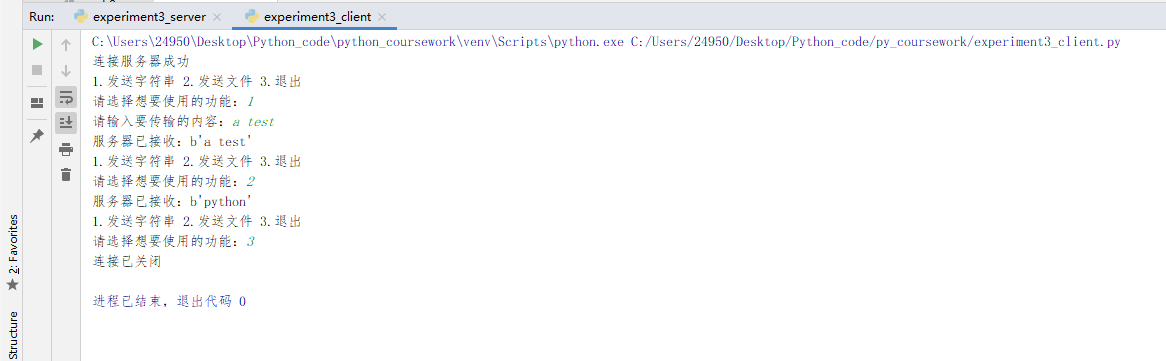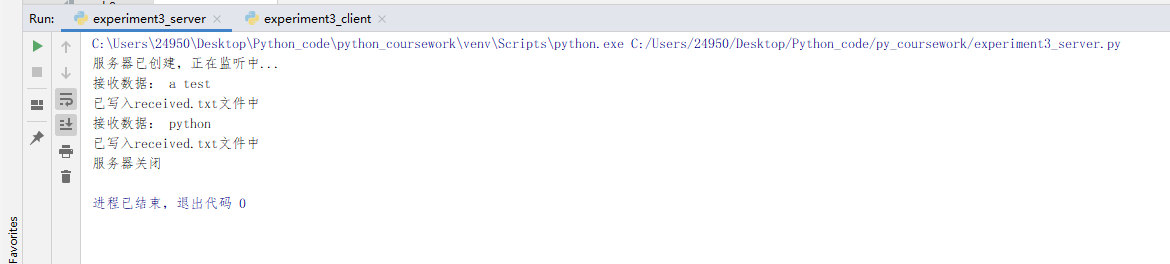# 20162120 2019-2020-2 《Python程序设计》实验三报告

## 1.实验内容

（1）创建服务端和客户端，选择一个通信端口，用Python语言编程实现通信演示程序；
（2）要求包含文件的基本操作，例如打开和读写操作。
（3）要求发送方从文件读取内容，加密后并传输；接收方收到密文并解密，保存在文件中。
（4）程序代码托管到码云。

## 2. 实验过程及结果

### （2）主要代码介绍

• 加解密部分：
基于pyDes库实现DES加解密功能。
from pyDes import des, CBC, PAD_PKCS5
import binascii
key_ = '12345675'
def des_encrypt(s):
"""
DES 加密
:param s: 原始字符串
:return: 加密后字符串，16进制
"""
secret_key = key_
iv = secret_key
k = des(secret_key, CBC, iv, pad=None, padmode=PAD_PKCS5)
en = k.encrypt(s, padmode=PAD_PKCS5)
return binascii.b2a_hex(en)
def des_descrypt(s):
"""
DES 解密
:param s: 加密后的字符串，16进制
:return:  解密后的字符串
"""
secret_key = key_
iv = secret_key
k = des(secret_key, CBC, iv, pad=None, padmode=PAD_PKCS5)
de = k.decrypt(binascii.a2b_hex(s), padmode=PAD_PKCS5)
return de

• 客户端部分：
循环发送字符串给服务器。取'close_'作为关闭连接的保留字。
import socket
from mydes import des_descrypt, des_encrypt
from tkinter.filedialog import askopenfilename
if __name__ == '__main__':
s = socket.socket(socket.AF_INET, socket.SOCK_STREAM)
s.connect(('127.0.0.1', 8001))
print('连接服务器成功')
while True:
ch = input('1.发送字符串 2.发送文件 3.退出\n请选择想要使用的功能：')
if ch == '1':
str_ = input('请输入要传输的内容：')
elif ch == '2':
filename = askopenfilename()
with open(filename, 'r', encoding='utf-8') as f:
str_ = f.read()
elif ch == '3':
str_ = 'close_'
str_ = des_encrypt(str_)
s.sendall(str_)
break
else:
print('无该选项，请重新输入')
str_ = des_encrypt(str_)
# print(type(str_))
s.sendall(str_)
data = s.recv(1024)
data = des_descrypt(data)
print(data.decode())
s.close()
print('连接已关闭')

• 服务器部分：
循环接收客户端发送的数据，并写入文件中。取'close_'作为关闭连接的保留字。
import socket
from mydes import des_descrypt, des_encrypt

if __name__ == '__main__':
s = socket.socket(socket.AF_INET, socket.SOCK_STREAM)
s.bind(('127.0.0.1', 8001))
print('服务器已创建，正在监听中...')
s.listen()
conn, address = s.accept()
while True:
data = conn.recv(1024)
data = des_descrypt(data)
# print(data, type(data))
if data == b'close_':
break
else:
with open('received', 'wb') as f:
f.write(data)
str_ = '服务器已接收：'+str(data)
str_ = des_encrypt(str_.encode())
conn.sendall(str_)
print('接收数据：', data.decode(), '\n已写入received.txt文件中')
s.close()
print('服务器关闭')


### （4）程序结果

• 发送字符串：• 发送文件• 结束连接服务器：## 其他（感悟、思考等）

socket编程一直是自己没有踏足过的领域，在王志强老师的课上学到了socket编程的知识并实际运用，使我受益良多。

## 参考资料

posted @ 2020-05-16 12:19  稚晖  阅读(274)  评论(0编辑  收藏